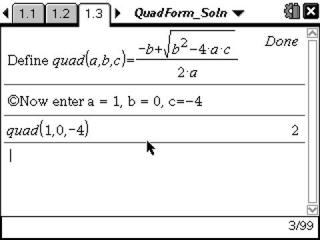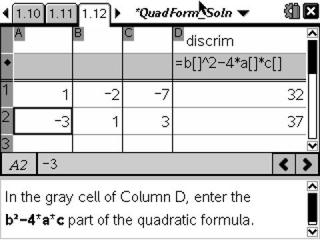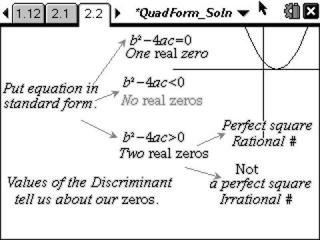# Activities

•##### Download

•• ##### Subject Area

• Math: Algebra I: Quadratic Functions
• Math: College Algebra: Equations and Inequalities
• Math: Developmental Mathematics - College: Radicals and Quadratic Equations

• ##### Author9-12

20 Minutes

• ##### Device
• TI-Nspire™ Navigator™
• TI-Nspire™
• TI-Nspire™ CAS
• ##### Software

TI-Nspire™
TI-Nspire™ CAS

2.0

• ##### Report an Issue

Quadratic Formula#### Activity Overview

Students make connections between the visual ways to find zeros of a parabola and algebraic ways with an emphasis on the quadratic formula.

#### Key Steps

•The student will utilize two defined functions to calculate the two solutions to quadratic functions. They will learn how to define the second quadratic (with the subtraction sign) themselves.

•Students will finally make the connection with the values for the discriminant, and how it is related to the type of solutions found for a quadratic function.

•A flow chart is presented to stimulate discussion that will tie the entire concept together. Students should discuss with a peer, and be able to make sketches of the four different scenarios.